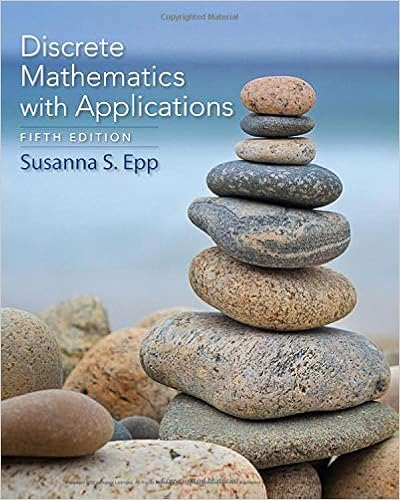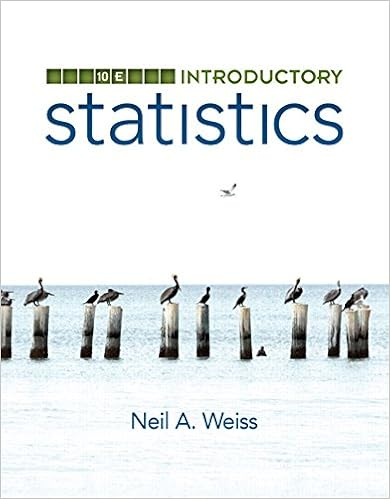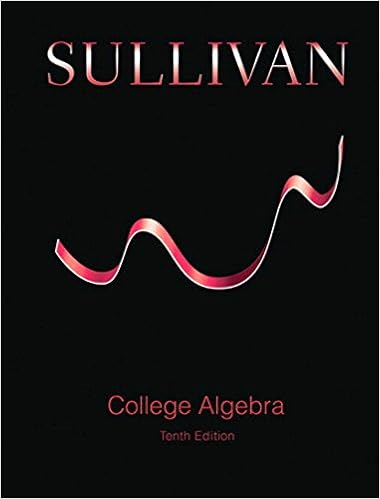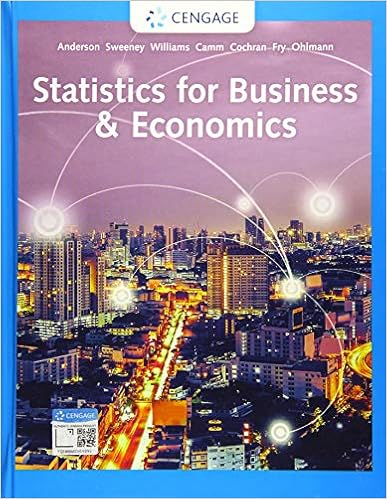# Extra-problems-week6.pdf - MATH 271 Summer 2016 Extra...

• 2

This preview shows page 1 - 2 out of 2 pages.

MATH 271 – Summer 2016Extra practice problems – Week 6University of CalgaryMark GirardHere are some questions to help you study graph theory for the final.1. (a) Draw a simple graph with exactly 4 vertices and 6 edges.(b) Draw a simple graph with exactly 6 vertices and 4 edges and exactly two connected components.(c) Draw a simple graph with exactly 8 vertices, one of which has degree 6.(d) Does there exist a graph with exactly 8 vertices, so that three of the vertices have degree 3 andthe remaining five vertices have degree 2? Explain.2. LetGbe the graphabdc(a) IsGbipartite?(b) Draw asimplegraphHwith exactly six verticesa, b, c, d, e, fand exactly seven edges and sothatGis a subgraph ofH.(c) Draw asimplegraphFwith exactly six verticesa, b, c, d, e, fso thatGis a subgraph ofFandFhas an Euler circuit.3. Consider the graphGgiven by:123456(a) IsGbipartite?(b) DoesGhave an Euler trail?(c) DoesGhave an Euler circuit?(d) DoesGhave a Hamiltonian circuit?4. Consider the graph given by:12345
##### We have textbook solutions for you!The document you are viewing contains questions related to this textbook.
Chapter 10 / Exercise 22
Discrete Mathematics With Applications
EppExpert VerifiedBrowse all Textbook Solutions

Upload your study docs or become a

Course Hero member to access this document

End of preview. Want to read all 2 pages?

Upload your study docs or become a

Course Hero member to access this document

Term
Winter
Professor
staff
Tags
Math, Vertex, Glossary of graph theory, Leonhard Euler, Eulerian path, Seven Bridges of K nigsberg
##### We have textbook solutions for you!
The document you are viewing contains questions related to this textbook.The document you are viewing contains questions related to this textbook.
Chapter 10 / Exercise 22
Discrete Mathematics With Applications
EppExpert Verified
•••